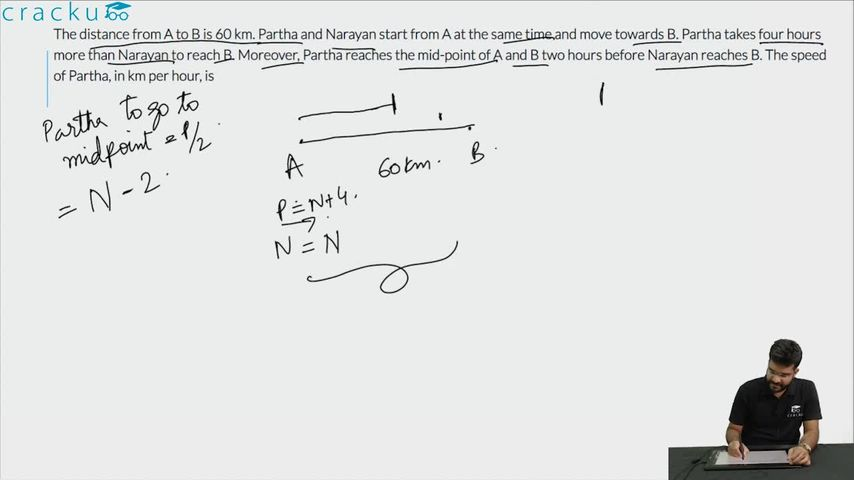Question 93

# The distance from A to B is 60 km. Partha and Narayan start from A at the same time and move towards B. Partha takes four hours more than Narayan to reach B. Moreover, Partha reaches the mid-point of A and B two hours before Narayan reaches B. The speed of Partha, in km per hour, is

Solution

Let the time taken by Partha to cover 60 km be x hours.
Narayan will cover 60 km in x-4 hours.

Speed of Partha = $$\frac{60}{x}$$
Speed of Narayan = $$\frac{60}{x-4}$$

Partha reaches the mid-point of A and B two hours before Narayan reaches B.

=> $$\dfrac{30}{\frac{60}{x}} + 2 = \dfrac{60}{\frac{60}{(x-4)}}$$

$$\frac{x}{2} + 2 = x-4$$
$$\frac{x+4}{2}=x-4$$
$$x+4=2x-8$$
$$x=12$$

Partha will take 12 hours to cross 60 km.
=> Speed of Partha = $$\frac{60}{12}=5$$ Kmph.
Therefore, option D is the right answer.

### View Video Solution